# Lecture 1: Playing KenKen

Playing KenKen is pretty simple: fill in the numbers 1-4 in the squares in the grid so that each row and column contains exactly one of each of the numbers from 1-4 with the restriction that each bolded area reach exactly the number given under the operation given in some order. You should be able to fill in the puzzle using only arithmetic and logic, without any guessing.

Let's start by trying to figure out this example of a KenKen puzzle to get a sense of the strategies involved. Here is an example puzzle: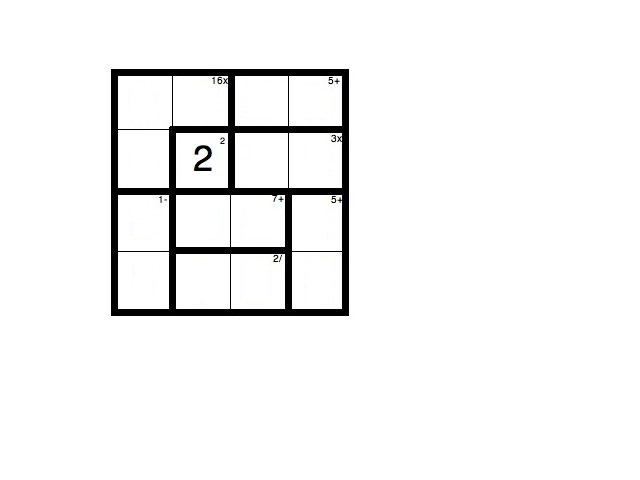We begin by noticing the free square with the 2 in it. Let's try starting near there. Notice the L-shaped bold area that should multiply to 16. We need to figure out all the possibilities of ways to multiply three of the numbers 1,2,3,4 together to get 16. There are only two ways to do this: 16=2*2*4=4*4*1. If we want to fit two of the same number in the L-shaped bold area we are given without violating the rule about no number appearing twice in each row and column then the repeated numbers have to be on the arms of the L, rather than one of them occupying the point of the L. This means that 2*2*4 is not a possibility for making 16, since then we would need to have two 2s in both the second row and second column which is against the rules. Therefore, we need to use 4*4*1=16. The puzzle so far looks like: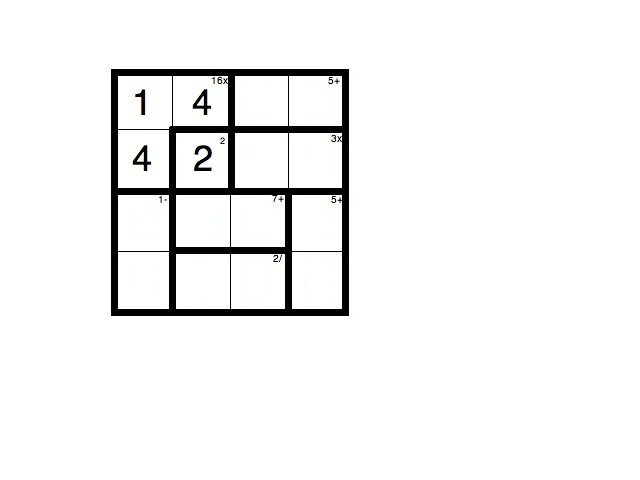Now let's look at the bolded rectangle that adds up to 7. The only way for two of the numbers 1,2,3,4 to add up to 7 is to use 3+4. Since we have already used 4 in the second column we can figure out which order the 3 and 4 go in in the rectangle and get the puzzle looking like: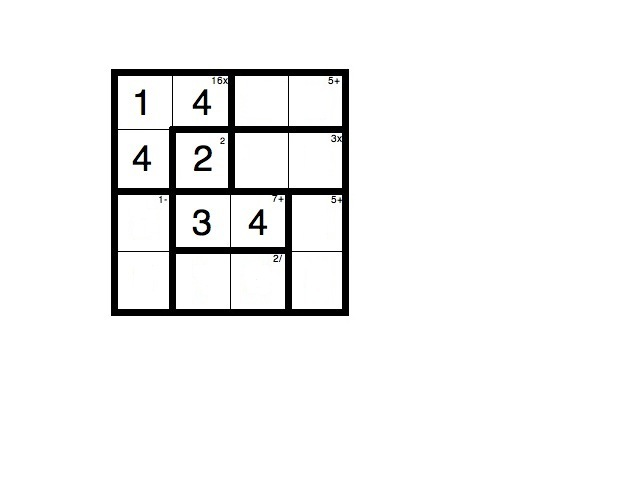Since we have filled in all but one of the numbers the in second column, we know that the last one must be a 1. Let's look at filling in the rest of that bold area which we must divide in some order to get two. In order to divide to get 2 we need to use either 2/1=2 or 4/2=2, but we already know that we have to use a 1 because one of the squares already has to be a 1, so we should fill in the other square in this bolded area with a 2. Now we get a puzzle that so far looks like: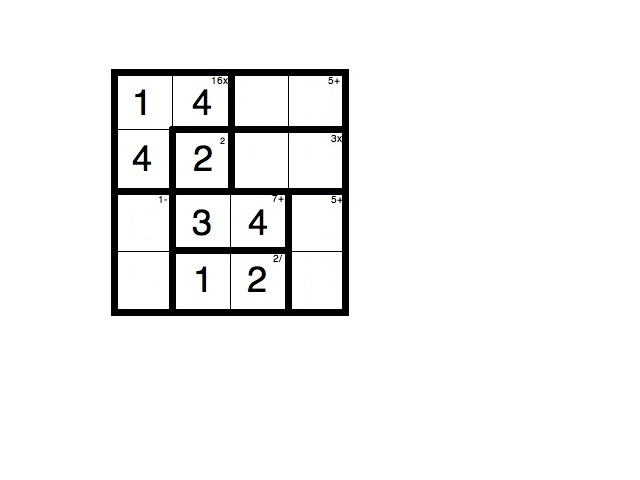the first row needs the numbers 3 and 2 to have exactly one of each of 1,2,3,4, which is good, since 3+2=5, which is what is supposed to happen. Since we can see that there is already a 2 in the third column, we can see that we have to fill them in with a 3 in the third column and a 2 in the fourth: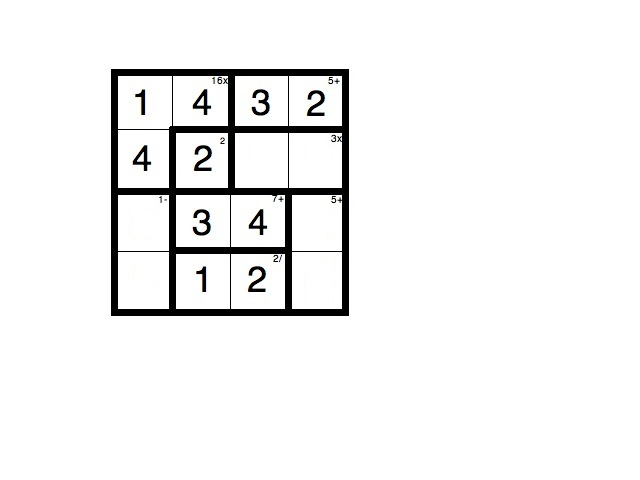We can now fill in the last number in the third column, since there is only one number missing from this column we can see that it should be the only one of 1,2,3,4 that hasn't yet appeared, which is a 1. The bolded rectangle that this 1 is in needs to multiply to be 3, so we know that the other entry in the rectangle must be a 3, to get: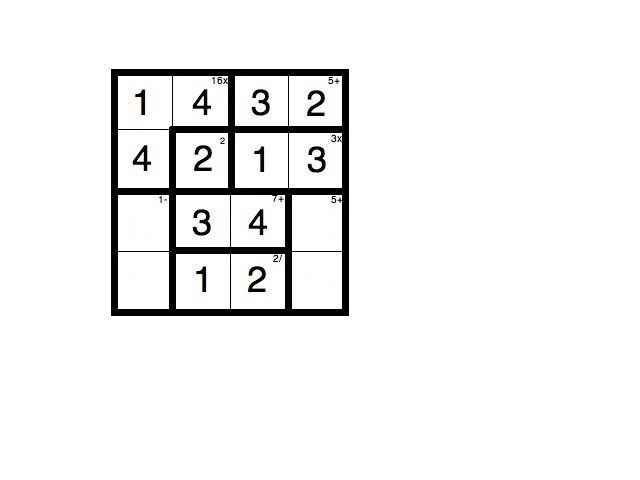The last column is only missing the numbers 1 and 4, so those must fill in the last two cells in that column. As a check we can see that they add up to 5, which is the requirement for that bolded area. Further, since the third row already contains a 4, we know that we need to fill in the 1 in the third row and the 4 in the fourth row. This leaves us with a puzzle with only one missing number in each of the last two rows: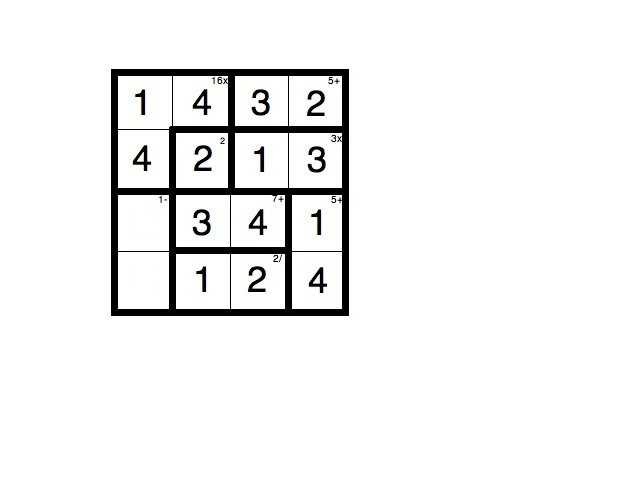Since there is only one number in each of the last two rows, we can fill it in, and check that they meet the arithmetic requirements, and get a final solution:There are a number of sources for more puzzles to play, in which you can play the 4x4 version that we just did an example of, plus 6x6 and even 8x8 versions in which you use the numbers 1 to 6 and 1 to 8 respectively, with the same rule that each number must appear exactly once in each row and column. Some places to find puzzles are:

• www.mathdoku.com
• www.kenken.com
• The New York Times website

This work was made possible through a grant from the National Science Foundation.